# Optimization (optimize)¶

There are several classical optimization algorithms provided by SciPy in the scipy.optimize package. An overview of the module is available using help (or pydoc.help):

from scipy import optimize
>>> info(optimize)
Optimization Tools
==================

A collection of general-purpose optimization routines.

(uses only function calls)
fmin_powell --  Powell's (modified) level set method (uses only
function calls)
fmin_cg     --  Non-linear (Polak-Ribiere) conjugate gradient algorithm
fmin_bfgs   --  Quasi-Newton method (Broydon-Fletcher-Goldfarb-Shanno);
fmin_ncg    --  Line-search Newton Conjugate Gradient (can use
leastsq     --  Minimize the sum of squares of M equations in
N unknowns given a starting estimate.

Constrained Optimizers (multivariate)

fmin_l_bfgs_b -- Zhu, Byrd, and Nocedal's L-BFGS-B constrained optimizer
(if you use this please quote their papers -- see help)

fmin_tnc      -- Truncated Newton Code originally written by Stephen Nash and
adapted to C by Jean-Sebastien Roy.

fmin_cobyla   -- Constrained Optimization BY Linear Approximation

Global Optimizers

anneal      --  Simulated Annealing
brute       --  Brute force searching optimizer

Scalar function minimizers

fminbound   --  Bounded minimization of a scalar function.
brent       --  1-D function minimization using Brent method.
golden      --  1-D function minimization using Golden Section method
bracket     --  Bracket a minimum (given two starting points)

Also a collection of general-purpose root-finding routines.

fsolve      --  Non-linear multi-variable equation solver.

Scalar function solvers

brentq      --  quadratic interpolation Brent method
brenth      --  Brent method (modified by Harris with hyperbolic
extrapolation)
ridder      --  Ridder's method
bisect      --  Bisection method
newton      --  Secant method or Newton's method

fixed_point --  Single-variable fixed-point solver.

A collection of general-purpose nonlinear multidimensional solvers.

broyden1            --  Broyden's first method - is a quasi-Newton-Raphson
method for updating an approximate Jacobian and then
inverting it
broyden2            --  Broyden's second method - the same as broyden1, but
broyden3            --  Broyden's second method - the same as broyden2, but
instead of directly computing the inverse Jacobian,
it remembers how to construct it using vectors, and
when computing inv(J)*F, it uses those vectors to
compute this product, thus avoding the expensive NxN
matrix multiplication.
broyden_generalized --  Generalized Broyden's method, the same as broyden2,
but instead of approximating the full NxN Jacobian,
it construct it at every iteration in a way that
avoids the NxN matrix multiplication.  This is not
as precise as broyden3.
anderson            --  extended Anderson method, the same as the
broyden_generalized, but added w_0^2*I to before
taking inversion to improve the stability
anderson2           --  the Anderson method, the same as anderson, but
formulated differently

Utility Functions

line_search --  Return a step that satisfies the strong Wolfe conditions.
check_grad  --  Check the supplied derivative using finite difference
techniques.


The first four algorithms are unconstrained minimization algorithms (fmin: Nelder-Mead simplex, fmin_bfgs: BFGS, fmin_ncg: Newton Conjugate Gradient, and leastsq: Levenburg-Marquardt). The last algorithm actually finds the roots of a general function of possibly many variables. It is included in the optimization package because at the (non-boundary) extreme points of a function, the gradient is equal to zero.

The simplex algorithm is probably the simplest way to minimize a fairly well-behaved function. The simplex algorithm requires only function evaluations and is a good choice for simple minimization problems. However, because it does not use any gradient evaluations, it may take longer to find the minimum. To demonstrate the minimization function consider the problem of minimizing the Rosenbrock function of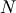variables: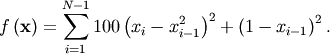The minimum value of this function is 0 which is achieved when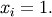This minimum can be found using the fmin routine as shown in the example below:

>>> from scipy.optimize import fmin
>>> def rosen(x):
...     """The Rosenbrock function"""
...     return sum(100.0*(x[1:]-x[:-1]**2.0)**2.0 + (1-x[:-1])**2.0)

>>> x0 = [1.3, 0.7, 0.8, 1.9, 1.2]
>>> xopt = fmin(rosen, x0, xtol=1e-8)
Optimization terminated successfully.
Current function value: 0.000000
Iterations: 339
Function evaluations: 571

>>> print xopt
[ 1.  1.  1.  1.  1.]


Another optimization algorithm that needs only function calls to find the minimum is Powell’s method available as fmin_powell.

## Broyden-Fletcher-Goldfarb-Shanno algorithm (fmin_bfgs)¶

In order to converge more quickly to the solution, this routine uses the gradient of the objective function. If the gradient is not given by the user, then it is estimated using first-differences. The Broyden-Fletcher-Goldfarb-Shanno (BFGS) method typically requires fewer function calls than the simplex algorithm even when the gradient must be estimated.

To demonstrate this algorithm, the Rosenbrock function is again used. The gradient of the Rosenbrock function is the vector: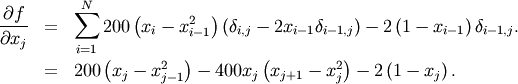This expression is valid for the interior derivatives. Special cases are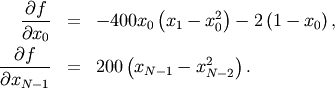A Python function which computes this gradient is constructed by the code-segment:

>>> def rosen_der(x):
...     xm = x[1:-1]
...     xm_m1 = x[:-2]
...     xm_p1 = x[2:]
...     der = zeros_like(x)
...     der[1:-1] = 200*(xm-xm_m1**2) - 400*(xm_p1 - xm**2)*xm - 2*(1-xm)
...     der = -400*x*(x-x**2) - 2*(1-x)
...     der[-1] = 200*(x[-1]-x[-2]**2)
...     return der


The calling signature for the BFGS minimization algorithm is similar to fmin with the addition of the fprime argument. An example usage of fmin_bfgs is shown in the following example which minimizes the Rosenbrock function.

>>> from scipy.optimize import fmin_bfgs

>>> x0 = [1.3, 0.7, 0.8, 1.9, 1.2]
>>> xopt = fmin_bfgs(rosen, x0, fprime=rosen_der)
Optimization terminated successfully.
Current function value: 0.000000
Iterations: 53
Function evaluations: 65
>>> print xopt
[ 1.  1.  1.  1.  1.]


The method which requires the fewest function calls and is therefore often the fastest method to minimize functions of many variables is fmin_ncg. This method is a modified Newton’s method and uses a conjugate gradient algorithm to (approximately) invert the local Hessian. Newton’s method is based on fitting the function locally to a quadratic form:where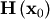is a matrix of second-derivatives (the Hessian). If the Hessian is positive definite then the local minimum of this function can be found by setting the gradient of the quadratic form to zero, resulting in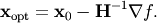The inverse of the Hessian is evaluted using the conjugate-gradient method. An example of employing this method to minimizing the Rosenbrock function is given below. To take full advantage of the NewtonCG method, a function which computes the Hessian must be provided. The Hessian matrix itself does not need to be constructed, only a vector which is the product of the Hessian with an arbitrary vector needs to be available to the minimization routine. As a result, the user can provide either a function to compute the Hessian matrix, or a function to compute the product of the Hessian with an arbitrary vector.

### Full Hessian example:¶

The Hessian of the Rosenbrock function is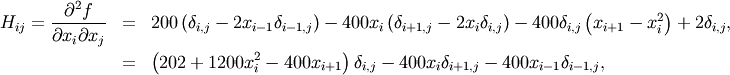if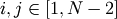with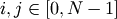defining the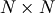matrix. Other non-zero entries of the matrix are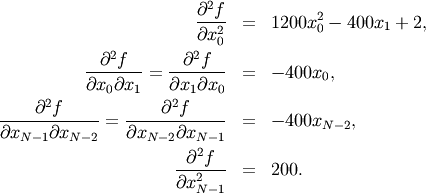For example, the Hessian when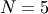is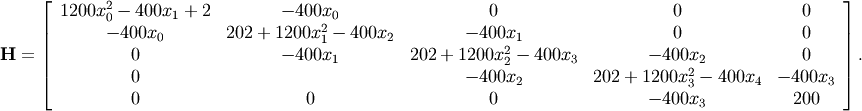The code which computes this Hessian along with the code to minimize the function using fmin_ncg is shown in the following example:

>>> from scipy.optimize import fmin_ncg
>>> def rosen_hess(x):
...     x = asarray(x)
...     H = diag(-400*x[:-1],1) - diag(400*x[:-1],-1)
...     diagonal = zeros_like(x)
...     diagonal = 1200*x-400*x+2
...     diagonal[-1] = 200
...     diagonal[1:-1] = 202 + 1200*x[1:-1]**2 - 400*x[2:]
...     H = H + diag(diagonal)
...     return H

>>> x0 = [1.3, 0.7, 0.8, 1.9, 1.2]
>>> xopt = fmin_ncg(rosen, x0, rosen_der, fhess=rosen_hess, avextol=1e-8)
Optimization terminated successfully.
Current function value: 0.000000
Iterations: 23
Function evaluations: 26
Hessian evaluations: 23
>>> print xopt
[ 1.  1.  1.  1.  1.]


### Hessian product example:¶

For larger minimization problems, storing the entire Hessian matrix can consume considerable time and memory. The Newton-CG algorithm only needs the product of the Hessian times an arbitrary vector. As a result, the user can supply code to compute this product rather than the full Hessian by setting the fhess_p keyword to the desired function. The fhess_p function should take the minimization vector as the first argument and the arbitrary vector as the second argument. Any extra arguments passed to the function to be minimized will also be passed to this function. If possible, using Newton-CG with the hessian product option is probably the fastest way to minimize the function.

In this case, the product of the Rosenbrock Hessian with an arbitrary vector is not difficult to compute. If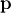is the arbitrary vector, then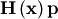has elements: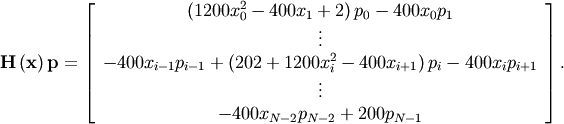Code which makes use of the fhess_p keyword to minimize the Rosenbrock function using fmin_ncg follows:

>>> from scipy.optimize import fmin_ncg
>>> def rosen_hess_p(x,p):
...     x = asarray(x)
...     Hp = zeros_like(x)
...     Hp = (1200*x**2 - 400*x + 2)*p - 400*x*p
...     Hp[1:-1] = -400*x[:-2]*p[:-2]+(202+1200*x[1:-1]**2-400*x[2:])*p[1:-1] \
...                -400*x[1:-1]*p[2:]
...     Hp[-1] = -400*x[-2]*p[-2] + 200*p[-1]
...     return Hp

>>> x0 = [1.3, 0.7, 0.8, 1.9, 1.2]
>>> xopt = fmin_ncg(rosen, x0, rosen_der, fhess_p=rosen_hess_p, avextol=1e-8)
Optimization terminated successfully.
Current function value: 0.000000
Iterations: 22
Function evaluations: 25
Hessian evaluations: 54
>>> print xopt
[ 1.  1.  1.  1.  1.]


## Least-square fitting (leastsq)¶

All of the previously-explained minimization procedures can be used to solve a least-squares problem provided the appropriate objective function is constructed. For example, suppose it is desired to fit a set of data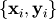to a known model,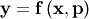whereis a vector of parameters for the model that need to be found. A common method for determining which parameter vector gives the best fit to the data is to minimize the sum of squares of the residuals. The residual is usually defined for each observed data-point as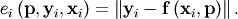An objective function to pass to any of the previous minization algorithms to obtain a least-squares fit is.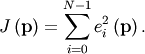The leastsq algorithm performs this squaring and summing of the residuals automatically. It takes as an input argument the vector function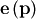and returns the value ofwhich minimizes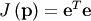directly. The user is also encouraged to provide the Jacobian matrix of the function (with derivatives down the columns or across the rows). If the Jacobian is not provided, it is estimated.

An example should clarify the usage. Suppose it is believed some measured data follow a sinusoidal pattern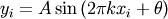where the parameters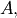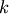, and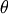are unknown. The residual vector is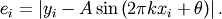By defining a function to compute the residuals and (selecting an appropriate starting position), the least-squares fit routine can be used to find the best-fit parameters. This is shown in the following example:

>>> from numpy import *
>>> x = arange(0,6e-2,6e-2/30)
>>> A,k,theta = 10, 1.0/3e-2, pi/6
>>> y_true = A*sin(2*pi*k*x+theta)
>>> y_meas = y_true + 2*random.randn(len(x))

>>> def residuals(p, y, x):
...     A,k,theta = p
...     err = y-A*sin(2*pi*k*x+theta)
...     return err

>>> def peval(x, p):
...     return p*sin(2*pi*p*x+p)

>>> p0 = [8, 1/2.3e-2, pi/3]
>>> print array(p0)
[  8.      43.4783   1.0472]

>>> from scipy.optimize import leastsq
>>> plsq = leastsq(residuals, p0, args=(y_meas, x))
>>> print plsq
[ 10.9437  33.3605   0.5834]

>>> print array([A, k, theta])
[ 10.      33.3333   0.5236]

>>> import matplotlib.pyplot as plt
>>> plt.plot(x,peval(x,plsq),x,y_meas,'o',x,y_true)
>>> plt.title('Least-squares fit to noisy data')
>>> plt.legend(['Fit', 'Noisy', 'True'])
>>> plt.show()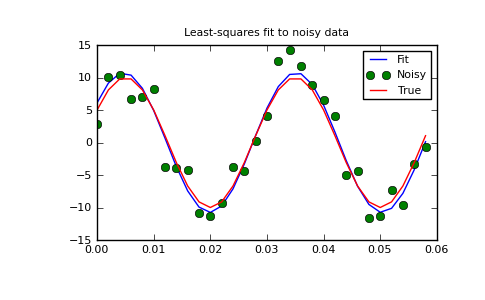## Sequential Least-square fitting with constraints (fmin_slsqp)¶

This module implements the Sequential Least SQuares Programming optimization algorithm (SLSQP).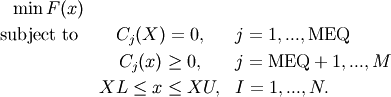The following script shows examples for how constraints can be specified.

"""
This script tests fmin_slsqp using Example 14.4 from Numerical Methods for
Engineers by Steven Chapra and Raymond Canale.  This example maximizes the
function f(x) = 2*x*y + 2*x - x**2 - 2*y**2, which has a maximum at x=2,y=1.
"""

from scipy.optimize import fmin_slsqp
from numpy import array, asfarray, finfo,ones, sqrt, zeros

def testfunc(d,*args):
"""
Arguments:
d     - A list of two elements, where d represents x and
d represents y in the following equation.
sign - A multiplier for f.  Since we want to optimize it, and the scipy
optimizers can only minimize functions, we need to multiply it by
-1 to achieve the desired solution
Returns:
2*x*y + 2*x - x**2 - 2*y**2

"""
try:
sign = args
except:
sign = 1.0
x = d
y = d
return sign*(2*x*y + 2*x - x**2 - 2*y**2)

def testfunc_deriv(d,*args):
""" This is the derivative of testfunc, returning a numpy array
representing df/dx and df/dy

"""
try:
sign = args
except:
sign = 1.0
x = d
y = d
dfdx = sign*(-2*x + 2*y + 2)
dfdy = sign*(2*x - 4*y)
return array([ dfdx, dfdy ],float)

from time import time

print '\n\n'

print "Unbounded optimization. Derivatives approximated."
t0 = time()
x = fmin_slsqp(testfunc, [-1.0,1.0], args=(-1.0,), iprint=2, full_output=1)
print "Elapsed time:", 1000*(time()-t0), "ms"
print "Results",x
print "\n\n"

print "Unbounded optimization.  Derivatives provided."
t0 = time()
x = fmin_slsqp(testfunc, [-1.0,1.0], args=(-1.0,), iprint=2, full_output=1)
print "Elapsed time:", 1000*(time()-t0), "ms"
print "Results",x
print "\n\n"

print "Bound optimization.  Derivatives approximated."
t0 = time()
x = fmin_slsqp(testfunc, [-1.0,1.0], args=(-1.0,),
eqcons=[lambda x, y: x-x ], iprint=2, full_output=1)
print "Elapsed time:", 1000*(time()-t0), "ms"
print "Results",x
print "\n\n"

print "Bound optimization (equality constraints).  Derivatives provided."
t0 = time()
x = fmin_slsqp(testfunc, [-1.0,1.0], fprime=testfunc_deriv, args=(-1.0,),
eqcons=[lambda x, y: x-x ], iprint=2, full_output=1)
print "Elapsed time:", 1000*(time()-t0), "ms"
print "Results",x
print "\n\n"

print "Bound optimization (equality and inequality constraints)."
print "Derivatives provided."

t0 = time()
x = fmin_slsqp(testfunc,[-1.0,1.0], fprime=testfunc_deriv, args=(-1.0,),
eqcons=[lambda x, y: x-x ],
ieqcons=[lambda x, y: x-.5], iprint=2, full_output=1)
print "Elapsed time:", 1000*(time()-t0), "ms"
print "Results",x
print "\n\n"

def test_eqcons(d,*args):
try:
sign = args
except:
sign = 1.0
x = d
y = d
return array([ x**3-y ])

def test_ieqcons(d,*args):
try:
sign = args
except:
sign = 1.0
x = d
y = d
return array([ y-1 ])

print "Bound optimization (equality and inequality constraints)."
print "Derivatives provided via functions."
t0 = time()
x = fmin_slsqp(testfunc, [-1.0,1.0], fprime=testfunc_deriv, args=(-1.0,),
f_eqcons=test_eqcons, f_ieqcons=test_ieqcons,
iprint=2, full_output=1)
print "Elapsed time:", 1000*(time()-t0), "ms"
print "Results",x
print "\n\n"

def test_fprime_eqcons(d,*args):
try:
sign = args
except:
sign = 1.0
x = d
y = d
return array([ 3.0*(x**2.0), -1.0 ])

def test_fprime_ieqcons(d,*args):
try:
sign = args
except:
sign = 1.0
x = d
y = d
return array([ 0.0, 1.0 ])

print "Bound optimization (equality and inequality constraints)."
print "Derivatives provided via functions."
print "Constraint jacobians provided via functions"
t0 = time()
x = fmin_slsqp(testfunc,[-1.0,1.0], fprime=testfunc_deriv, args=(-1.0,),
f_eqcons=test_eqcons, f_ieqcons=test_ieqcons,
fprime_eqcons=test_fprime_eqcons,
fprime_ieqcons=test_fprime_ieqcons, iprint=2, full_output=1)
print "Elapsed time:", 1000*(time()-t0), "ms"
print "Results",x
print "\n\n"


## Scalar function minimizers¶

Often only the minimum of a scalar function is needed (a scalar function is one that takes a scalar as input and returns a scalar output). In these circumstances, other optimization techniques have been developed that can work faster.

### Unconstrained minimization (brent)¶

There are actually two methods that can be used to minimize a scalar function (brent and golden), but golden is included only for academic purposes and should rarely be used. The brent method uses Brent’s algorithm for locating a minimum. Optimally a bracket should be given which contains the minimum desired. A bracket is a triple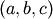such that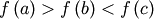and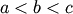. If this is not given, then alternatively two starting points can be chosen and a bracket will be found from these points using a simple marching algorithm. If these two starting points are not provided 0 and 1 will be used (this may not be the right choice for your function and result in an unexpected minimum being returned).

### Bounded minimization (fminbound)¶

Thus far all of the minimization routines described have been unconstrained minimization routines. Very often, however, there are constraints that can be placed on the solution space before minimization occurs. The fminbound function is an example of a constrained minimization procedure that provides a rudimentary interval constraint for scalar functions. The interval constraint allows the minimization to occur only between two fixed endpoints.

For example, to find the minimum of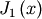near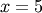, fminbound can be called using the interval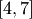as a constraint. The result is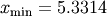:

>>> from scipy.special import j1
>>> from scipy.optimize import fminbound
>>> xmin = fminbound(j1, 4, 7)
>>> print xmin
5.33144184241


## Root finding¶

### Sets of equations¶

To find the roots of a polynomial, the command roots is useful. To find a root of a set of non-linear equations, the command fsolve is needed. For example, the following example finds the roots of the single-variable transcendental equation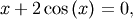and the set of non-linear equations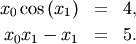The results are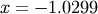and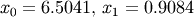.

>>> def func(x):
...     return x + 2*cos(x)

>>> def func2(x):
...     out = [x*cos(x) - 4]
...     out.append(x*x - x - 5)
...     return out

>>> from scipy.optimize import fsolve
>>> x0 = fsolve(func, 0.3)
>>> print x0
-1.02986652932

>>> x02 = fsolve(func2, [1, 1])
>>> print x02
[ 6.50409711  0.90841421]


### Scalar function root finding¶

If one has a single-variable equation, there are four different root finder algorithms that can be tried. Each of these root finding algorithms requires the endpoints of an interval where a root is suspected (because the function changes signs). In general brentq is the best choice, but the other methods may be useful in certain circumstances or for academic purposes.

### Fixed-point solving¶

A problem closely related to finding the zeros of a function is the problem of finding a fixed-point of a function. A fixed point of a function is the point at which evaluation of the function returns the point: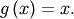Clearly the fixed point of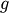is the root of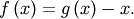Equivalently, the root of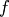is the fixed_point of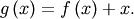The routine fixed_point provides a simple iterative method using Aitkens sequence acceleration to estimate the fixed point ofgiven a starting point.